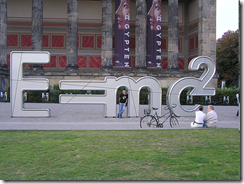## Blog

### The Einstein's formula proven but it is just outdated

It has been very successful on digg, the post on the practicaldemonstration of Einstein's famous formula E=mc^2. In about two days almost 3000 times Dugg.
Actually, the experimental demonstration of Einstein's formula was given directly and indirectly thousands of times. Every day in particle accelerators Scientists are studying nuclear phenomena using the Einstein's formula and particle accelerators itself are designed taken into consideration it.
What I wanted to bring to your attention is that in fact Einstein's formula was to be about 7 years included in a more general context, valid at higher energies, in technical terms known as the Planck Energy.
In this context, the Theory of Special Relativity is an approximation of a general theory, valid at high energies, and would be valid at the lower energies currently measurable as an approximation.

But what new theory are we talking about?
We're talking about a new relativistic theory outlined by 2 Italian Physicists and a Polish, based on non-commutative geometry. According to this theory, the geometry at high energies is not as smooth as generally tought the space-time continuous  but could not be continue and therefore quantum. The purpose of this new theory would be to reconcile, at Planck energy, the Theory of Gravity (The Einstein's General Relativity) with the Quantum Theory of the Fundamental Interactions.
The results are presented in a famous paper published in 2001 on Physics Letters B, one of the most important scientific journals in physics, and followed by a multitude of papers that have inspired to it. I report here the title and the abstract:

We derive finite boost transformations based on the Lorentz sector of the bicross-product-basis $\kappa$-Poincare' Hopf albegra. We emphasize the role of these boost transformations in a recently-proposed new relativistic theory. We find that when the (dimensionful) deformation parameter is identified with the Planck length, which together with the speed-of-light constant has the status of observer-independent scale in the new relativistic theory, the deformed boosts saturate at the value of momentum that corresponds to the inverse of the Planck length.